Electric Circuits - Lesson 4 - Circuit Connections

# Parallel Circuits

As mentioned in a previous section of Lesson 4, two or more electrical devices in a circuit can be connected by series connections or by parallel connections. When all the devices are connected using parallel connections, the circuit is referred to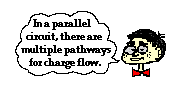as a parallel circuit. In a parallel circuit, each device is placed in its own separate branch. The presence of branch lines means that there are multiple pathways by which charge can traverse the external circuit. Each charge passing through the loop of the external circuit will pass through a single resistor present in a single branch. When arriving at the branching location or node, a charge makes a choice as to which branch to travel through on its journey back to the low potential terminal.

A short comparison and contrast between series and parallel circuits was made in an earlier section of Lesson 4. In that section, it was emphasized that the act of adding more resistors to a parallel circuit results in the rather unexpected result of having less overall resistance. Since there are multiple pathways by which charge can flow, adding another resistor in a separate branch provides another pathway by which to direct charge through the main area of resistance within the circuit. This decreased resistance resulting from increasing the number of branches will have the effect of increasing the rate at which charge flows (also known as the current). In an effort to make this rather unexpected result more reasonable, a tollway analogy was introduced. A tollbooth is the main location of resistance to car flow on a tollway. Adding additional tollbooths within their own branch on a tollway will provide more pathways for cars to flow through the toll station. These additional tollbooths will decrease the overall resistance to car flow and increase the rate at which they flow.

### Current

The rate at which charge flows through a circuit is known as the current. Charge does NOT pile up and begin to accumulate at any given location such that the current at one location is more than at other locations. Charge does NOT become used up by resistors in such a manner that there is less current at one location compared to another. In a parallel circuit, charge divides up into separate branches such that there can be more current in one branch than there is in another. Nonetheless, when taken as a whole, the total amount of current in all the branches when added together is the same as the amount of current at locations outside the branches. The rule that current is everywhere the same still works, only with a twist. The current outside the branches is the same as the sum of the current in the individual branches. It is still the same amount of current, only split up into more than one pathway.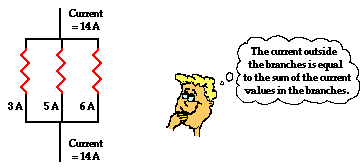In equation form, this principle can be written as

Itotal = I1 + I2 + I3 + ...

where Itotal is the total amount of current outside the branches (and in the battery) and I1, I2, and I3 represent the current in the individual branches of the circuit.

Throughout this unit, there has been an extensive reliance upon the analogy between charge flow and water flow. Once more, we will return to the analogy to illustrate how the sum of the current values in the branches is equal to the amount outside of the branches. The flow of charge in wires is analogous to the flow of water in pipes. Consider the diagrams below in which the flow of water in pipes becomes divided into separate branches. At each node (branching location), the water takes two or more separate pathways. The rate at which water flows into the node (measured in gallons per minute) will be equal to the sum of the flow rates in the individual branches beyond the node. Similarly, when two or more branches feed into a node, the rate at which water flows out of the node will be equal to the sum of the flow rates in the individual branches that feed into the node.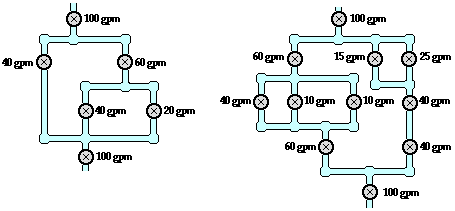The same principle of flow division applies to electric circuits. The rate at which charge flows into a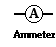node is equal to the sum of the flow rates in the individual branches beyond the node. This is illustrated in the examples shown below. In the examples a new circuit symbol is introduced - the letter A enclosed within a circle. This is the symbol for an ammeter - a device used to measure the current at a specific point. An ammeter is capable of measuring the current while offering negligible resistance to the flow of charge.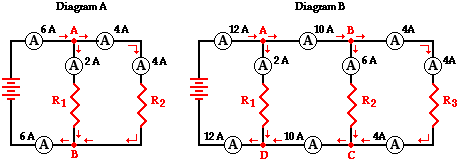Diagram A displays two resistors in parallel with nodes at point A and point B. Charge flows into point A at a rate of 6 amps and divides into two pathways - one through resistor 1 and the other through resistor 2. The current in the branch with resistor 1 is 2 amps and the current in the branch with resistor 2 is 4 amps. After these two branches meet again at point B to form a single line, the current again becomes 6 amps. Thus we see the principle that the current outside the branches is equal to the sum of the current in the individual branches holds true.

Itotal = I1 + I2

6 amps = 2 amps + 4 amps

Diagram B above may be slightly more complicated with its three resistors placed in parallel. Four nodes are identified on the diagram and labeled A, B, C and D. Charge flows into point A at a rate of 12 amps and divides into two pathways - one passing through resistor 1 and the other heading towards point B (and resistors 2 and 3). The 12 amps of current is divided into a 2 amp pathway (through resistor 1) and a 10 amp pathway (heading toward point B). At point B, there is further division of the flow into two pathways - one through resistor 2 and the other through resistor 3. The current of 10 amps approaching point B is divided into a 6-amp pathway (through resistor 2) and a 4-amp pathway (through resistor 3). Thus, it is seen that the current values in the three branches are 2 amps, 6 amps and 4 amps and that the sum of the current values in the individual branches is equal to the current outside the branches.

Itotal = I1 + I2 + I3

12 amps = 2 amps + 6 amps + 4 amps

A flow analysis at points C and D can also be conducted and it is observed that the sum of the flow rates heading into these points is equal to the flow rate that is found immediately beyond these points.

### Equivalent Resistance

The actual amount of current always varies inversely with the amount of overall resistance. There is a clear relationship between the resistance of the individual resistors and the overall resistance of the collection of resistors. To explore this relationship, let's begin with the simplest case of two resistors placed in parallel branches, each having the same resistance value of 4 Ω. Since the circuit offers two equal pathways for charge flow, only one-half the charge will choose to pass through a given branch. While each individual branch offers 4 Ω of resistance to any charge that flows through it, only one-half of all the charge flowing through the circuit will encounter the 4 Ω resistance of that individual branch. Thus, as far as the battery that is pumping the charge is concerned, the presence of two 4-Ω resistors in parallel would be equivalent to having one 2-Ω resistor in the circuit. In the same manner, the presence of two 6-Ω resistors in parallel would be equivalent to having one 3-Ω resistor in the circuit. And the presence of two 12-Ω resistors in parallel would be equivalent to having one 6-Ω resistor in the circuit.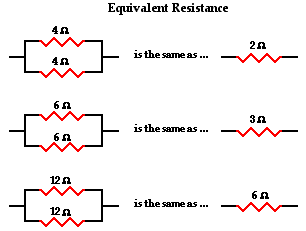Now let's consider another simple case of having three resistors in parallel, each having the same resistance of 6 Ω. With three equal pathways for charge to flow through the external circuit, only one-third the charge will choose to pass through a given branch. Each individual branch offers 6 Ω of resistance to the charge that passes through it. However, the fact that only one-third of the charge passes through a particular branch means that the overall resistance of the circuit is 2 Ω. As far as the battery that is pumping the charge is concerned, the presence of three 6-Ω resistors in parallel would be equivalent to having one 2-Ω resistor in the circuit. In the same manner, the presence of three 9-Ω resistors in parallel would be equivalent to having one 3-Ω resistor in the circuit. And the presence of three 12-Ω resistors in parallel would be equivalent to having one 4-Ω resistor in the circuit.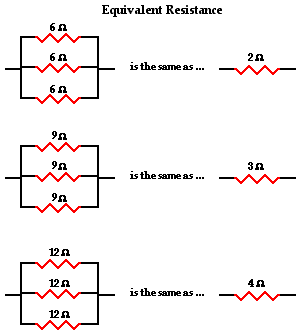This is the concept of equivalent resistance. The equivalent resistance of a circuit is the amount of resistance that a single resistor would need in order to equal the overall effect of the collection of resistors that are present in the circuit. For parallel circuits, the mathematical formula for computing the equivalent resistance (Req) is

1 / Req = 1 / R1 + 1 / R2 + 1 / R3 + ...

where R1, R2, and R3 are the resistance values of the individual resistors that are connected in parallel. The examples above could be considered simple cases in which all the pathways offer the same amount of resistance to an individual charge that passes through it. The simple cases above were done without the use of the equation. Yet the equation fits both the simple cases where branch resistors have the same resistance values and the more difficult cases where branch resistors have different resistance values. For instance, consider the application of the equation to the one simple and one difficult case below.

 Case 1: Three 12 Ω resistors are placed in parallel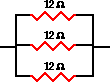1/Req = 1/R1 + 1/R2 + 1/R3 1/Req = 1/(12 Ω) + 1/(12 Ω) + 1/(12 Ω) Using a calculator ... 1/Req = 0.25 Ω-1 Req = 1 / (0.25 Ω-1) Req = 4.0 Ω Case 2: A 5.0 Ω, 7.0 Ω, and 12 Ω resistor are placed in parallel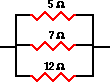1/Req = 1/R1 + 1/R2 + 1/R3 1/Req = 1/(5.0 Ω) + 1/(7.0 Ω) + 1/(12 Ω) Using a calculator ... 1/Req = 0.42619 Ω-1 Req = 1 / (0.42619 Ω-1) Req = 2.3 Ω

## It's Your Turn to Try It

Need more practice? Use the Two Resistors in Parallel widget below to try some additional problems. Enter any two resistance values you wish. Use your calculator to determine the values of Req. Then click the Submit button to check your answers. Try it as many times as you wish with different resistance values.

### Voltage Drops for Parallel Branches

It has been emphasized throughout the Circuits unit of The Physics Classroom tutorial that whatever voltage boost is acquired by a charge in the battery is lost by the charge as it passes through the resistors of the external circuit. The total voltage drop in the external circuit is equal to the gain in voltage as a charge passes through the internal circuit. In a parallel circuit, a charge does not pass through every resistor; rather, it passes through a single resistor. Thus, the entire voltage drop across that resistor must match the battery voltage. It matters not whether the charge passes through resistor 1, resistor 2, or resistor 3, the voltage drop across the resistor that it chooses to pass through must equal the voltage of the battery. Put in equation form, this principle would be expressed as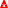Vbattery =V1 =V2 =V3 = ...

If three resistors are placed in parallel branches and powered by a 12-volt battery, then the voltage drop across each one of the three resistors is 12 volts. A charge flowing through the circuit would only encounter one of these three resistors and thus encounter a single voltage drop of 12 volts.

Electric potential diagrams were introduced in Lesson 1 of this unit and subsequently used to illustrate the consecutive voltage drops occurring in series circuits. An electric potential diagram is a conceptual tool for representing the electric potential difference between several points on an electric circuit. Consider the circuit diagram below and its corresponding electric potential diagram.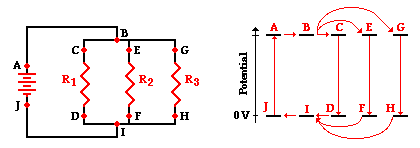As shown in the electric potential diagram, positions A, B, C, E and G are all at a high electric potential. A single charge chooses only one of the three possible pathways; thus at position B, a single charge will move towards point C, E or G and then passes through the resistor that is in that branch. The charge does not lose its high potential until it passes through the resistor, either from C to D, E to F, or G to H. Once it passes through a resistor, the charge has returned to nearly 0 Volts and returns to the negative terminal of the battery to obtain its voltage boost. Unlike in series circuits, a charge in a parallel circuit encounters a single voltage drop during its path through the external circuit.

The current through a given branch can be predicted using the Ohm's law equation and the voltage drop across the resistor and the resistance of the resistor. Since the voltage drop is the same across each resistor, the factor that determines that resistor has the greatest current is the resistance. The resistor with the greatest resistance experiences the lowest current and the resistor with the least resistance experiences the greatest current. In this sense, it could be said that charge (like people) chooses the path of least resistance. In equation form, this could be stated as

 I1 = ΔV1 / R1 I2 = ΔV2 / R2 I3 = ΔV3 / R3

This principle is illustrated by the circuit shown below. The product of I•R is the same for each resistor (and equal to the battery voltage). Yet the current is different in each resistor. The current is greatest where the resistance is least and the current is least where the resistance is greatest.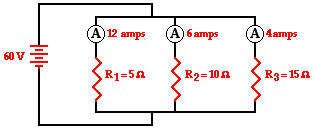### Mathematical Analysis of Parallel Circuits

The above principles and formulae can be used to analyze a parallel circuit and determine the values of the current at and electric potential difference across each of the resistors in a parallel circuit. Their use will be demonstrated by the mathematical analysis of the circuit shown below. The goal is to use the formulae to determine the equivalent resistance of the circuit (Req), the current through the battery (Itot), and the voltage drops and current for each of the three resistors.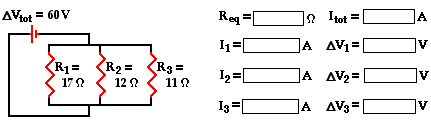The analysis begins by using the resistance values for the individual resistors in order to determine the equivalent resistance of the circuit.

1 / Req = 1 / R1 + 1 / R2 + 1 / R3 = (1 / 17 Ω) + (1 / 12 Ω) + (1 / 11 Ω)

1 / Req = 0.23306 Ω-1

Req = 1 / (0.23306 Ω-1)

Req = 4.29 Ω

(rounded from 4.29063 Ω)

Now that the equivalent resistance is known, the current in the battery can be determined using the Ohm's law equation. In using the Ohm's law equation (ΔV = I • R) to determine the current in the battery, it is important to use the battery voltage for ΔV and the equivalent resistance for R. The calculation is shown here:

Itot = ΔVbattery / Req = (60 V) / (4.29063 Ω)

Itot = 14.0 amp

(rounded from 13.98396 amp)

The 60 V battery voltage represents the gain in electric potential by a charge as it passes through the battery. The charge loses this same amount of electric potential for any given pass through the external circuit. That is, the voltage drop across each one of the three resistors is the same as the voltage gained in the battery:

ΔV battery = ΔV1 = ΔV2 = ΔV3 = 60 V

There are three values left to be determined - the current in each of the individual resistors. Ohm's law is used once more to determine the current values for each resistor - it is simply the voltage drop across each resistor (60 Volts) divided by the resistance of each resistor (given in the problem statement). The calculations are shown below.

 I1 = ΔV1 / R1 I1 = (60 V) / (17 Ω) I1 = 3.53 amp I2 = ΔV 2 / R2 I2 = (60 V) / (12 Ω) I2 = 5.00 amp I3 = ΔV 3 / R3 I3 = (60 V) / (11 Ω) I3 = 5.45 amp

As a check of the accuracy of the mathematics performed, it is wise to see if the calculated values satisfy the principle that the sum of the current values for each individual resistor is equal to the total current in the circuit (or in the battery). In other words, is Itot = I1 + I2 + I3 ?

Is Itot = I1 + I2 + I3 ?

Is 14.0 amp = 3.53 amp + 5.00 amp + 5.45 amp ?

Is 14.0 amp = 13.98 amp ?

Yes!!

(The 0.02 amp difference is simply the result of having previously rounded the Itot value from 13.98.)

The mathematical analysis of this parallel circuit involved a blend of concepts and equations. As is often the case in physics, the divorcing of concepts from equations when embarking on the solution to a physics problem is a dangerous act. Here, one must consider the concepts that the voltage drops across each one of the three resistors is equal to the battery voltage and that the sum of the current in each resistor is equal to the total current. These understandings are essential in order to complete the mathematical analysis. In the next part of Lesson 4, combination or compound circuits in which some devices are in parallel and others are in series will be investigated.

### More Practice

Make, solve and check your own problems by using the Equivalent Resistance widget below. Make yourself a problem with any number of resistors and any values. Solve the problem; then click on the Submit button to check your answer.

### We Would Like to Suggest ...Why just read about it and when you could be interacting with it? Interact - that's exactly what you do when you use one of The Physics Classroom's Interactives. We would like to suggest that you combine the reading of this page with the use of our DC Circuit Builder Interactive. You can find it in the Physics Interactives section of our website. The DC Circuit Builder provides the learner with a virtual circuit building kit. You can easily drag voltage sources, resistors and wires onto the workspace and arrange and connect them anyway you wish. Voltmeters and ammeters allow you to measure current and voltage drops. Tapping a resistor or a voltage source allows you to change the resistance or the input voltage. It's easy. It's fun. And it's safe (unless you're using it in the bathtub).

### Check Your Understanding

1. As more and more resistors are added in parallel to a circuit, the equivalent resistance of the circuit ____________ (increases, decreases) and the total current of the circuit ____________ (increases, decreases).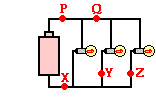2. Three identical light bulbs are connected to a D-cell as shown below. P, Q, X, Y and Z represent locations along the circuit. Which one of the following statements is true?

a. The current at Y is greater than the current at Q.

b. The current at Y is greater than the current at P.

c. The current at Y is greater than the current at Z.

d. The current at P is greater than the current at Q.

e. The current at Q is greater than the current at P.

f. The current is the same at all locations.3. Three identical light bulbs are connected to a D-cell as shown below. P, Q, X, Y and Z represent locations along the circuit. At which location(s), if any, will the current be ...

a. ... the same as at X?

b. ... the same as at Q?

c. ... the same as at Y?

d. ... less than at Q?

e. ... less than at P?

f. ... twice that at Z?

g. ... three times that at Y?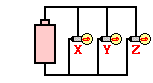4. Which adjustments could be made to the circuit below that would decrease the current in the cell? List all that apply.

a. Increase the resistance of bulb X.

b. Decrease the resistance of bulb X.

c. Increase the resistance of bulb Z.

d. Decrease the resistance of bulb Z.

e. Increase the voltage of the cell (somehow).

f. Decrease the voltage of the cell (somehow).

g. Remove bulb Y.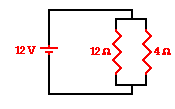5. A 12-V battery , a 12-ohm resistor and a 4-ohm resistor are connected as shown. The current in the 12-ohm resistor is ____ that in the 4-ohm resistor.

 a. 1/3 b. 1/2 c. 2/3 d. the same as e. 1.5 times f. twice g. three times h. four times6. A 12-V battery , a 12-ohm resistor and a 4-ohm resistor are connected as shown. The voltage drop across the 12-ohm resistor is ____ that across the 4-ohm resistor.

 a. 1/3 b. 1/2 c. 2/3 d. the same as e. 1.5 times f. twice g. three times h. four times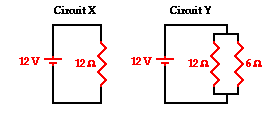7. A 12-V battery and a 12-ohm resistor are connected as shown in circuit. A 6-ohm resistor is added to the 12-ohm resistor to create circuit Y as shown. The voltage drop across the 6-ohm resistor in circuit Y is ____ that across the resistor in X.

a. larger than

b. smaller than

c. the same as

8. Use your understanding of equivalent resistance to complete the following statements:

a. Two 6-Ω resistors placed in parallel would provide a resistance that is equivalent to one _____-Ω resistor.

b. Three 6-Ω resistors placed in parallel would provide a resistance that is equivalent to one _____-Ω resistor.

c. Three 8-Ω resistors placed in parallel would provide a resistance that is equivalent to one _____-Ω resistor.

d. Three resistors with resistance values of 2-Ω, 4-Ω, and 6-Ω are placed in parallel. These would provide a resistance that is equivalent to one _____-Ω resistor.

e. Three resistors with resistance values of 5-Ω, 6-Ω, and 7-Ω are placed in parallel. These would provide a resistance that is equivalent to one _____-Ω resistor.

f. Three resistors with resistance values of 12-Ω, 6-Ω, and 21-Ω are placed in parallel. These would provide a resistance that is equivalent to one _____-Ω resistor.

9. Based on your answers to the above question, complete the following statement:

The overall or equivalent resistance of three resistors placed in parallel will be _____.

a. greater than the resistance of the biggest R value of the three.

b. less than the resistance of the smallest R value of the three.

c. somewhere in between the smallest R and the biggest R value of the three.

d. ... nonsense! No such generalization can be made. The results vary.

10. Three resistors are connected in parallel. If placed in a circuit with a 12-volt power supply. Determine the equivalent resistance, the total circuit current, and the voltage drop across and current in each resistor.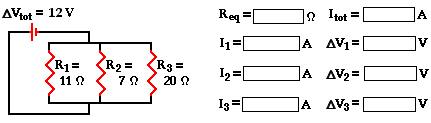Next Section:

Follow Us